HOME / Applications / Thermo-structural behavior of RF Microwave power divider

# Thermo-structural behavior of RF Microwave power divider

View 3D results Used Tools:## Introduction

Radio frequency (RF) and microwave systems typically require a perfect power distribution networks performed through two types of microwave structures: Dividers to split an input signal into two or more output signals and Combiners to associate RF input signals from multiple inputs into single RF output signal. These structures are indispensable in high-power applications like accelerators, radars, and heating systems which need high accuracy power splitting (With necessary amplitude and phase distribution at the outputs) and low operating power losses.

At high power average level, waveguide-based structures are commonly used for a wide variety of multi-port power dividers/combiners. Such technology is applicable to power distribution networks due to its inherent advantages in power handling capacity and signal integrity with the characteristic of low power loss at high frequencies.
There are many classes of waveguide-based power dividers: T-type, Y-type, E-type, magic Tee waveguide (WG) junction, etc. The studied WG power splitter, proposed in this study, is the Folded H plane tee junction. It is a three-ports divider in which its output and input arms are implemented in a single plane along a single axis, thus taking less space in the overall system.  The next figure is showing the working prototype of the analyzed splitter.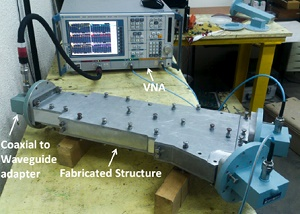Figure 1 - Working prototype of the proposed WG power divider 

## Problem description

The proposed Waveguide power divider is made of 3 ports. It allows to split the input power into two equi-phasic and equi-amplitude signal outputs. The 3D geometrical structure of the outer copper housing with its air cavity are shown in figure 2. It is constituted of 4 sections: the first unit section S1 is made of a standard rectangular waveguide WR-284 depending on the operational frequency (3.7 GHz in our case to ensure the dominant mode of propagation), a second tapered section S2 followed by a straight waveguide section S3 giving birth to two equal output arms. The geometrical dimensions of each section are detailed in table 1.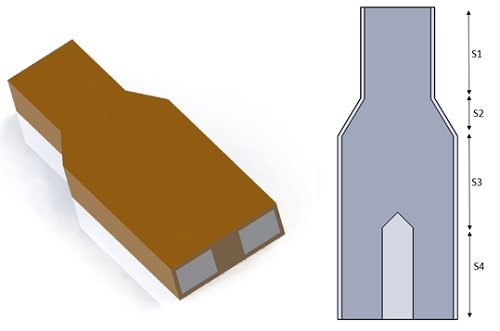Figure 2 - The 3D design of the studied power divider

Table 1 - Geometrical dimensions
 Section Dimension (mm) Section S1 width=72.136 height=34.036 Length=100 Section S2 width=121 height=34.036 Length=40.54 Section S3 width=121 height=34.036 Length=100 Section S4 (Arm1,2) width=43.659 height=34.036 Length=100

## Simulation Setup

The S-parameters solver of HFWorks is used, coupled to the thermal and structural cases for a working frequency range of [3.45GHz-3.9GHz]. The properties of the used materials are summarized in table 2.

Table 2 - Material properties
 Material Relative permittivity Dielectric loss tangent Electrical conductivity (S/m) Thermal conductivity (W/m.K) Thermal expansion coefficient (1/K) Elastic modulus (GPa) Poisson`s ratio Copper 1 0 5.96E+7 401 1.6 E-5 110 0.343

### Electromagnetic boundary conditions

Wave port: The wave port boundary is applied to the input and outputs cavity ports.

### Thermal boundary conditions

For an excitation power ofapplied to the input port, a thermal boundary convection is applied to the inner faces of copper housing at an ambient temperature of 20°C and a convection coefficient set to.

### Structural boundary conditions

A fixed boundary conditions are applied to the lateral faces of the copper housing corresponding to the input and outputs ports.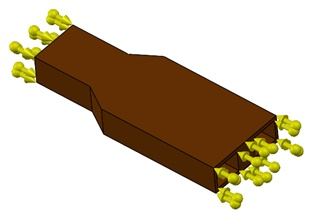Figure 3 - Applied mechanical boundary conditions

## Mesh

To enhance the accuracy of the obtained results, a fine mesh control was applied to the studied model like shown in the next figure of the meshed model.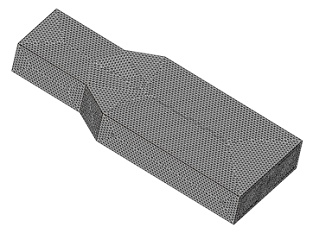Figure 4 - Meshed model

## Results

A fast sweep S-parameters study for the frequency range of [3.45GHz-3.9GHz] revealed the next results for a resonant frequency around 3.7 GHz:

The electric field distribution versus phase is presented by the next figure. It is showing an excellent power division at 3.7GHz.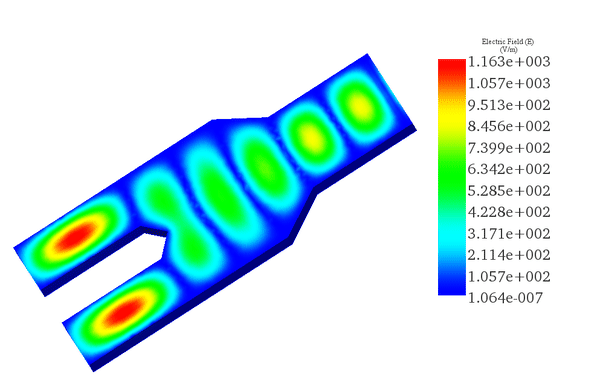Figure 5 - Electric Field animation versus phase.

The frequency response of the splitter is depicted in the next 2D plot of the return and insertion losses (S11-S21) results. It shows an acceptable response under -20dB for a bandwidth of 140 MHz centered around 3.7GHz.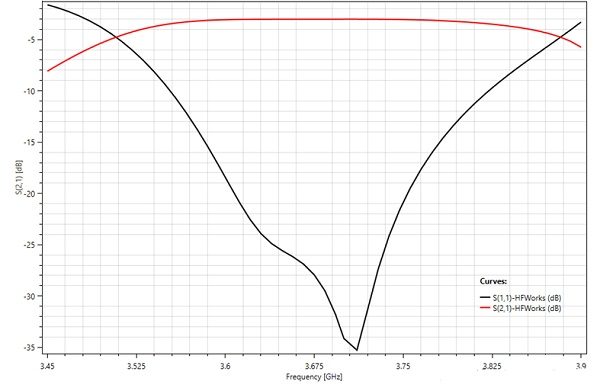Figure 6 - Return and insertion loss results versus frequency

## Thermal results

HFWorks comes with an integrated Thermal Solver. It allows to analyze the thermal behavior of your High frequency design by computing the temperature, temperature gradient, and heat flux due to the thermal loads caused by the conductor losses in our case.

A steady state thermal analysis of the power splitter was performed at the resonant frequency of 3.7GHz. For an input power excitation of 500kW applied to the input port at an ambient temperature of 20°C and under the mentioned thermal boundary conditions, the simulation gives the next results:

The temperature distribution across the metallic housing is shown by figure 7; it achieves a maximum value of 573°C crosswise the output arms. This temperature rise cannot be tolerated in the structure; it affects the safety of its handling and  reduces its electromagnetic performance.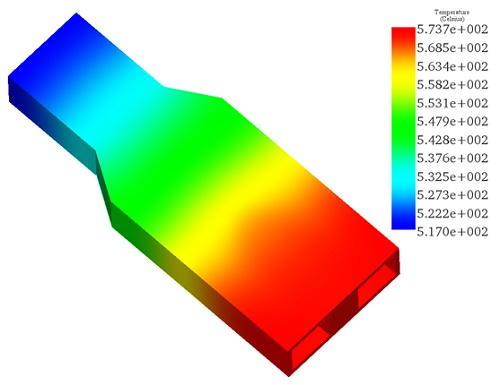Figure 7 - Temperature distribution at 3.7GHz

## Structural results

The Thermal solver feeds the thermal loads to the Structural solver which computes the final stress and displacements that affects the electromagnetic behavior of the splitter while considering the applied structural constraints.

The next figure is showing the thermal Von Mises stress distribution across the metallic part. the maximum stress obtained at certain points is 3.72 E+9 N/m²  and the maximum deformation is up to 1.2mm.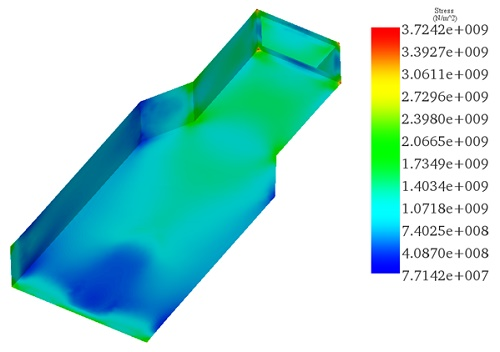Figure 8 - Von Mises stress distribution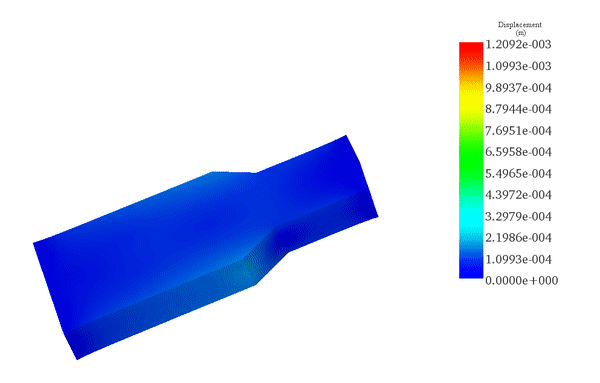Figure 9 - Mechanical displacement animation

Even with small deformations, if it is permanent, it can degrade the S-parameters of the structure and reduces its life-time after repeated uses. As such, a forced convection cooling is required to cool down the power divider and keeps its fully efficiency for a long working cycle.

## Conclusion

Thermal and structural management of RF power divider/combiner is extremely required at high average power level. It allows to avoid the induced thermal stresses that affects their structure and then limits their effective service life. For this example, a forced convection cooling allows to reduce the temperature rise of the structure to a value ten times lower than the non-cooled case, according to the reference . Such solution helps to improve the power handling capacity of these waveguide-based components.

#### References

. Dixit, Harish V., et al. "Design data for quick development of folded H plane tee at high average power level." S?dhan?43.3 (2018): 33.
View 3D model and 3D results